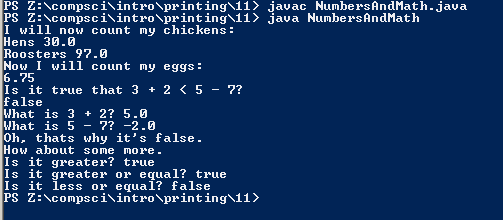# Assignemnt #11, NumbersAndMath

## Code

```    /// Name: Carlos Sosa
/// Period: 6
/// Date: 9/25/15
/// Program Name: NumbersAndMath
/// File Name: NumbersAndMath.java
public class NumbersAndMath
{
public static void main( String[] args )
{
System.out.println( "I will now count my chickens:" );
System.out.println( "Hens " + ( 25.0 + 30.0 / 6.0) );
/// % takes the percent of the first number of the second number
System.out.println( "Roosters " + ( 100.0 - 25.0 * 3.0 % 4.0 ) );

System.out.println( "Now I will count my eggs:" );
/// Adds, subtracts,devides and takes percentages
System.out.println( 3.0 + 2.0 + 1.0 - 5.0 + 4.0 % 2.0 - 1.0 / 4.0 + 6.0 );

System.out.println( "Is it true that 3 + 2 < 5 - 7?" );
/// Calculates if the statement is true or false
System.out.println( 3.0 + 2.0 < 5.0 - 7.0 );
System.out.println( "What is 3 + 2? " + ( 3.0 + 2.0 ) );
System.out.println( "What is 5 - 7? " + ( 5.0 - 7.0 ) );

System.out.println( "Oh, thats why it's false." );

System.out.println( "How about some more." );
/// Calculates greater than, less than, greater than or equal to, less than or equal too
System.out.println( "Is it greater? " + ( 5.0 > -2.0 ) );
System.out.println( "Is it greater or equal? " + ( 5.0 >= -2.0 ) );
System.out.println( "Is it less or equal? " + ( 5.0 <= -2.0 ) );
}
}
```

### Picture of the output